Courses

# Chemistry Test 3 - Atomic Structure, Mole Concept, Chemical Bonding, Periodic Properties, States Of Matter, Equilibrium, Hydrocarbons, General Organic Compounds, Alkyl Halide

## 30 Questions MCQ Test JEE Main Mock Test Series 2020 & Previous Year Papers | Chemistry Test 3 - Atomic Structure, Mole Concept, Chemical Bonding, Periodic Properties, States Of Matter, Equilibrium, Hydrocarbons, General Organic Compounds, Alkyl Halide

Description
This mock test of Chemistry Test 3 - Atomic Structure, Mole Concept, Chemical Bonding, Periodic Properties, States Of Matter, Equilibrium, Hydrocarbons, General Organic Compounds, Alkyl Halide for JEE helps you for every JEE entrance exam. This contains 30 Multiple Choice Questions for JEE Chemistry Test 3 - Atomic Structure, Mole Concept, Chemical Bonding, Periodic Properties, States Of Matter, Equilibrium, Hydrocarbons, General Organic Compounds, Alkyl Halide (mcq) to study with solutions a complete question bank. The solved questions answers in this Chemistry Test 3 - Atomic Structure, Mole Concept, Chemical Bonding, Periodic Properties, States Of Matter, Equilibrium, Hydrocarbons, General Organic Compounds, Alkyl Halide quiz give you a good mix of easy questions and tough questions. JEE students definitely take this Chemistry Test 3 - Atomic Structure, Mole Concept, Chemical Bonding, Periodic Properties, States Of Matter, Equilibrium, Hydrocarbons, General Organic Compounds, Alkyl Halide exercise for a better result in the exam. You can find other Chemistry Test 3 - Atomic Structure, Mole Concept, Chemical Bonding, Periodic Properties, States Of Matter, Equilibrium, Hydrocarbons, General Organic Compounds, Alkyl Halide extra questions, long questions & short questions for JEE on EduRev as well by searching above.
QUESTION: 1

### IUPAC name of compound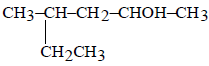is

Solution: The functional group is OH and it should get low locant and longest chain should be selected for naming so methane becomes substituient and get locant number 4
QUESTION: 2

Solution:
QUESTION: 3

### The nature of inter-molecular forces among benzene molecules is

Solution: Intermolecular Forces - Benzene. There are three different types of intermolecular forces, dipole interaction, hydrogen bonding, and dispersion forces. The only one that occurs with Benzene is dispersion forces because it is a non-polar molecule.
QUESTION: 4
The geometrical isomerism is shown
Solution:
QUESTION: 5

The addition of HCN to a carbonyl compound is an example of

Solution: As carbonyl contains pi bond which broke and later get added therefore it is a ncleophilic addtion reaction.
QUESTION: 6
Acetaldehyde is the rearrangement product of
Solution:
QUESTION: 7
In the presence of platinum catalyst, hydrocarbon A adds hydrogen to form n-hexane. When hydrogen bromide is added to A instead of hydrogen, only a single bromo compound is formed. Which of the following is A ?
Solution:
QUESTION: 8
Which of the following will convert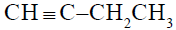to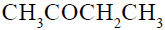?
Solution:
QUESTION: 9

1,3-Butadiene when treated with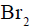gives

Solution:
QUESTION: 10
The alkene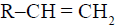reacts readily with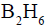and formed the product B which on oxidation with alkaline hydrogen peroxides produces
Solution:
QUESTION: 11
The ortho-para directing group among the following is
Solution:
QUESTION: 12
Amongst the following, the compound that can be most readily sulphonated is
Solution:
−CH3 group that has +1 effect and due to its presence, toluene has highest electron density in the benzene ring.
For this reason Toluene can be easily sulphonated.
QUESTION: 13
Chloroform on warming with Ag powder gives
Solution:
QUESTION: 14

Ethyl alcohol gives ethyl chloride with the help of

Solution:

With SOCl2, alcohols give Haloalkanes the best way and also from NaCl we obtain only Cl- which is weak nucleophile and cannot replace OH- which is a stronger nucleophile.

QUESTION: 15
Which of the following compound will make precipitate most readily with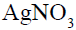?
Solution:
QUESTION: 16
Which of the following is responsible for depletion of the ozone layer in the upper strata of the atmosphere?
Solution:
QUESTION: 17
7.5 grams of a gas occupy 5.6 litres of volume at STP. The gas is
Solution:
QUESTION: 18
The number of water molecules present in a drop of water (volume 0.0018 ml) at room temperature is
Solution: The density of water is 1g/cm3 Volume of a drop of water =0.0018ml Mass of water drop=0.0018g Moles of water=0.0001 No of water molecules in 1 drop =0.0001X6.022X10^23 =6.022X10^19 water molecules.
QUESTION: 19
The correct representation of Charle’s law is given by
Solution:
QUESTION: 20
The structure of TlCl is similar to CsCl. What would be the radius ratio in TlCl ?
Solution:
QUESTION: 21
In the saphalerite (ZnS) structure,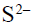ions form a face- centered cubic lattice. Then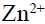ions are present on the body diagonals at
Solution:
QUESTION: 22
Which substance shows anti-ferromagnetism ?
Solution:
QUESTION: 23
An electron has principal quantum number 3. The number of its (i) sub-shells and (ii) orbitals would be respectively
Solution: Here principal quantum number =3 as the values of azimuthal quantum number = 0 to n-1 so the number of Subshells = 0,1,2(s p d) as per the formula, number of orbitals in a given principal quantum number = n^2 where n=principal quantum number so the answer is 3 and 9
QUESTION: 24
Which of the following molecule is linear ?
Solution:
QUESTION: 25

The number of water molecules present in a drop of water (volume 0.0018 ml) at room temperature is

Solution:
QUESTION: 26
Heat of neutralisation is least when
Solution:
QUESTION: 27
Starting with one mole of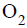and two moles of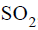the equilibrium for the formation of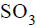was established at a certain temperature. If V is the volume of the vessel and 2x is the number of moles ofpresent, the equilibrium constant will be
Solution:
QUESTION: 28
The atomic numbers of other elements which lie in the same group as the tenth element in the periodic table are
Solution:
QUESTION: 29
A hydride of nitrogen which as acidic is
Solution:
QUESTION: 30
Which one of the following removes temporary hardness of water ?
Solution: Explanation: There are a number of methods to remove the hardness present in water. When any of these methods are followed, hard water gets converted into soft water. Some of the methods to remove hardness from water are: 1. Chemical Process of Boiling Hard Water. 2. Adding Slaked Lime (Clark's Process). 3. Adding Washing Soda 4. Calgon Process 5. Ion Exchange Process 6. Using Ion Exchange Resins. Hence, in above given question we can remove the temporary hardness of water by adding slaked lime.